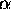# Ohm's Law Prelab Assignment

Name:_______________________________ 4-digit Code Number:_______________________________

Re-read Taylor Error Analysis, Chapters 1, 2, 3, and 4.

1. A certain resistor is made of a material for which= 0.01/oC. When placed in a mixture of ice and water, its resistance is determined to be 150 ohms. What is the resistance if the resistor is placed in boiling water?
2. Consider the two circuits in the lab manual (cct1 and cct2) containing a voltmeter and an ammeter. Why is circuit 1 preferable (smaller error) if the unknown resistor R is large, whereas circuit 2 is preferable if R is small?
3. What precautions should be noted before measuring resistance with the digital multimeter? To what setting should the function switch be set? Into which two of the four jacks should the probe leads be inserted?
4. Write Ohm's law in equation form and explain what the equation means. Do not simply translate the symbols from math into English, but rather explain the content of the law.
5. List three methods for measuring resistance. Which is used in this experiment?
6. In the procedure for collecting data for the voltage vs. current plot, it was suggested that the current not exceed 1 amp. Why is it undesirable to use currents larger than this amount? Hint: Consider power dissipation and temperature.
7. Derive the error propagation equation (see Taylor, Chapters 1-3) for the uncertainty in resistance (R) given the uncertainties in voltage (V) and current (i).

Go to the lab